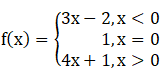If a function f: R → R be defined byFind: f (1), f (–1), f (0), f (2).

Asked by Sakshi | 1 year ago |  71

##### Solution :-

Given:

Let us find f (1), f (–1), f (0) and f (2).

When x > 0, f (x) = 4x + 1

Substituting x = 1 in the above equation, we get

f (1) = 4(1) + 1

= 4 + 1

= 5

When x < 0, f(x) = 3x – 2

Substituting x = –1 in the above equation, we get

f (–1) = 3(–1) – 2

= –3 – 2

= –5

When x = 0, f(x) = 1

Substituting x = 0 in the above equation, we get

f (0) = 1

When x > 0, f(x) = 4x + 1

Substituting x = 2 in the above equation, we get

f (2) = 4(2) + 1

= 8 + 1

= 9

∴ f (1) = 5, f (–1) = –5, f (0) = 1 and f (2) = 9.

Answered by Aaryan | 1 year ago

### Related Questions

#### Let R = {(a, b) : a, b, ϵ N and a < b}.Show that R is a binary relation on N

Let R = {(a, b) : a, b, ϵ N and a < b}.Show that R is a binary relation on N, which is neither reflexive nor symmetric. Show that R is transitive.

#### Let A = (1, 2, 3} and B = {4} How many relations can be defined from A to B.

Let A = (1, 2, 3} and B = {4} How many relations can be defined from A to B.

#### Let R = {(x, x2) : x is a prime number less than 10}.

Let R = {(x, x2) : x is a prime number less than 10}.

(i) Write R in roster form.

(ii) Find dom (R) and range (R).